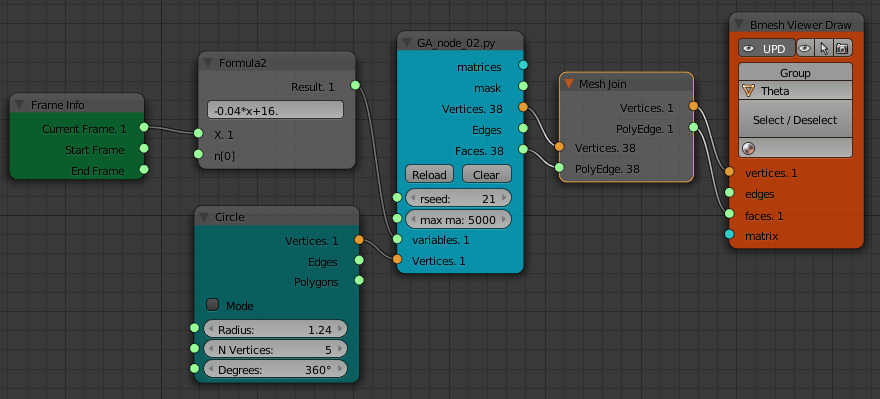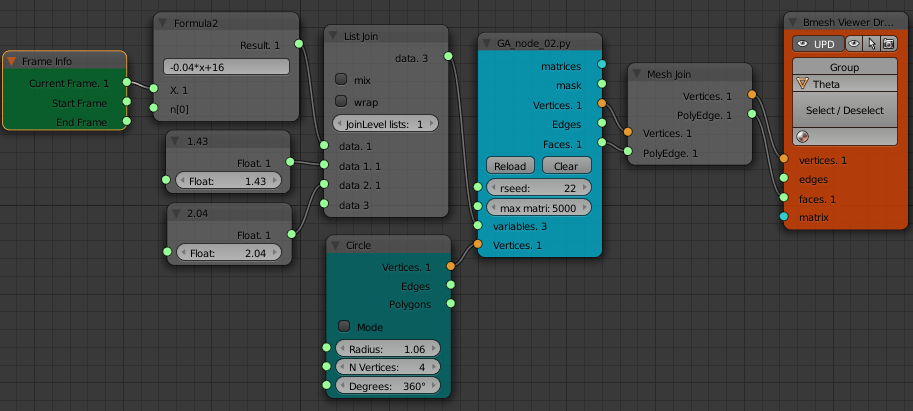# Structure Synth meets Sverchok - AnimationUpdate: This lsystem/Structure Synth code has now (March 2016) been incorporated into the Sverchok Generative Art node. See the updated examples and the node docs

In the previous two posts (Generative Art in Blender and Mesh Mode I introduced the Sverchok scripted node I'd written to implement Structure Synth generative art or lsystems inside Blender.

Following Martinius's suggestion I've now exposed parameters in the xml rule definition to the Sverchok node tree so we can do animation of Structure Synth lsystems inside Blender.

Here's an example:

As before, to use the generative art node in Blender first install the Sverchok addon. Download the lsystem code from github. Then load the three python files in the Blender directory (`LSystem_blender.py, GA_xml.py, GA_node.py`) as separate text blocks into a blend file. Add a "Scripted Node" to a Sverchok node tree. On the node select the `GA_node.py` code from the lower drop down. Then click the plugin icon to the right of this field. The node should turn blue with some inputs and outputs.

The latest version now has an additional input "variables".

The first step to animating the lsystem is to edit the lsystem definition in the xml text in `GA_xml.py` to define which values becomes variables. The value for any of the transforms in the file can be replaced with a pair of curly braces:

```transforms = "tx 0.5 rx 20 sa 0.9"
```

becomes

```transforms = "tx {} rx 20 sa 0.9"
```

To illustrate this here is the xml definition and the node diagram for the fern animation.

```Library["FernVars"] = """
<rules max_depth="2000">
<rule name="entry">
<call  rule="curl1" />
<call  rule="curl2" />
</rule>

<rule name="curl1" max_depth="60">
<call transforms="rx {} tz 1.5 s 0.9 0.9 1.0" rule="curl1"/>
<instance shape="box"/>
</rule>

<rule name="curl2" max_depth="40">
<call transforms="rx {} tz 1.5 s 0.9 0.9 1.0" rule="curl2"/>
<call transforms="tx 0.1 ty -0.45 ry 40 sa 0.25" rule="curlsmall" />
</rule>

<rule name="curlsmall" max_depth="40">
<call transforms="rx 2*{} tz 2.7 s 0.9 0.9 1.0" rule="curlsmall"/>
<instance shape="box"/>
</rule>
</rules>
"""
```For this animation the index number of the current frame in the animation is translated from the range 1 to 250 to the range 16 to 6 via the formula node and wired into the "variables" input of the "GA_node". This cause the fern to unwind as the animation proceeds. The separate objects are joined into one using the "Mesh Join" node and this output sent to the "Bmesh Viewer Draw" node.

If you want to animate more than one parameter use index numbers (starting with 0) inside the braces:

```transforms = "tx {0} rx {1} sa {2}"
```

With multiple parameters a "List Join" node is used to make a list to use as the "variables" input of the "GA_node".If not enough variables are provided the last one is repeated as many times as required.

Simple maths can also be use in the transforms definition. This has been used above in the "curlsmall" rule. The rx rotation of the transform will always be twice that of the rx rotation in the "curl1" and "curl2" rules.

There cannot be any spaces in any maths expressions for the rotation, translation or scale parameters when using a transforms attribute string as above. Instead of listing all the transforms in one attribute string each transform can now have its own string. This allows more complicated expressions to be included.

transforms as single attribute (no spaces allowed in maths expression)

```<call transforms="tx 1 rz -1*{0} ry {1}" rule="R1"/>
```

each transform with its own attribute (can have spaces)

```<call tx="1" rz="-1 * {0}" ry="{1}" rule="R1"/>
```

All this is implemented by first using python's string format method to substitute in the variable value from the node input. Then the resulting string is passed to python's `eval()` function. The string must evaluate to a single number (float or integer). Using `eval()` is a potential security problem as in theory someone could put some malicious code inside an xml lsystem definition. As always don't run code from a source you don't trust.

Only the transforms that take a single number that is tx, ty, tz, rx, ry, rz, sx, sy, sz and sa have been implemented using individual attributes. The ones that use triplets to specify all three translations or scales at once (t and s) can only be used in a transform string.

There's room to do some very clever stuff using this ability to put short bits of code in the xml...